### TIMEVALUE Function – Text to Time in Excel, VBA, G Sheets

This tutorial demonstrates how to use the Excel TIMEVALUE Function in Excel to convert a time stored as text into a valid time.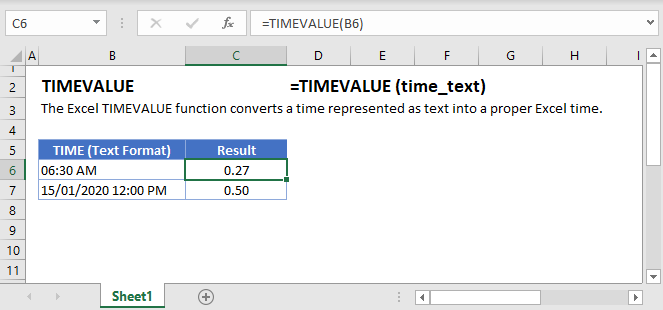## TIMEVALUE Function Overview

The TIMEVALUE Function Converts a time stored as text into the time’s corresponding serial number.

To use the TIMEVALUE Excel Worksheet Function, select a cell and type: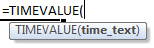(Notice how the formula inputs appear)

## TIMEVALUE Function Syntax and Inputs:

`=TIMEVALUE(time_text)`
AutoMacro - VBA Code Generator

### Function Arguments ( Inputs ):

time_text – The time stored as text. Example “6:00:00”

## Convert Time Stored as Text to Time

When working in spreadsheets you might encounter time stored as text.  You can usually identify times stored as text because the times are left-aligned and are proceeded by apostrophes: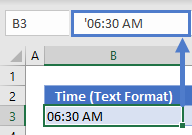These “times” are treated as text and you’ll be unable to work with them like normal times.

One way to convert the text to a date is with the TIMEVALUE Function:

`=TIMEVALUE(B3)`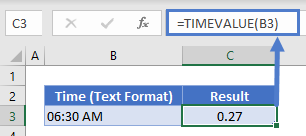### Things to know about the TIMEVALUE Function:

• TIMEVALUE ignores date information. To convert a date+time stored as text to a number, use the VALUE Function instead. Or use the DATEVALUE function to get the date only.

## TIMEVALUE in Google Sheets

The TIMEVALUE Function works exactly the same in Google Sheets as in Excel: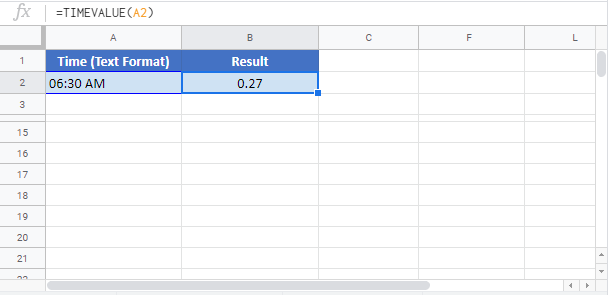## TIMEVALUE Examples in VBA

You can also use the TIMEVALUE function in VBA. Type:` application.worksheetfunction.timevalue(time_text)`

Running the following VBA statements

```Range("B2") = TimeValue(Range("A2"))
Range("B3") = TimeValue(Range("A3"))```

will create the following output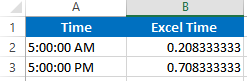For the function arguments (time_text, etc.), you can either enter them directly into the function, or define variables to use instead.

Return to the List of all Functions in Excel

## Excel Practice Worksheet

Practice Excel functions and formulas with our 100% free practice worksheets!

• Automatically Graded Exercises
• Learn Excel, Inside Excel!